# Test: Sale And Purchase- 2

## 20 Questions MCQ Test Quantitative Reasoning for GMAT | Test: Sale And Purchase- 2

Description
Attempt Test: Sale And Purchase- 2 | 20 questions in 40 minutes | Mock test for GMAT preparation | Free important questions MCQ to study Quantitative Reasoning for GMAT for GMAT Exam | Download free PDF with solutions
QUESTION: 1

### A furniture dealer sold two chairs for \$300 each. He made a profit of 25 percent on one of the chairs and a loss of 25 percent on theother. What was his overall loss or profit percentage on selling both the chairs?

Solution:

Given:

• Chair-1 selling price = \$300
• Profit% on selling chair-1 = 25%
• Chair-2 selling price = \$300
• Loss% on selling chair-2 = 25%

To Find: Overall profit or loss% on selling both chairs?

Approach: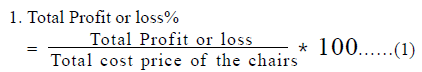• Total profit or loss = Profit on chair-1 – Loss on chair 2
• Total cost price of the chairs = Cost Price of chair-1 + Cost Price of chair-2

2. The cost price of the chairs can be found out using the information given about the selling price and the profit% or loss%

3. Cost Price and profit on chair-1

• Selling Price = Cost Price + Profit……..(2)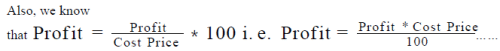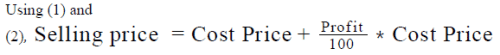As we know the selling price and the profit%, we can calculate the Cost price of chair-1. Once we know the cost price, we can calculate profit made on the chair using (2).

4. Cost Price and loss on chair-2
Selling Price = Cost Price - Loss……..(4)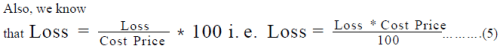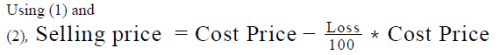As we know the selling price and the loss%, we can calculate the Cost price of chair-2. Once we know the cost price, we can calculate loss made on the chair using (4).
5. Now, as we know the cost price of the chairs and profit or loss made on the chair, we can calculate the profit or loss% using (1)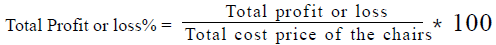Working out:

1. Cost Price and profit on chair-1

a. Selling price of the chair = \$300
b. Profit% on the chair = 25%
c. So, we can write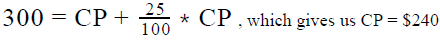2. Cost Price and loss on chair-2
a. Selling price of the chair = \$300
b. Loss% on the chair = 25%
c. So, we can write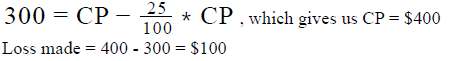3. As the loss made on chair-2 is greater than the profit made on chair-2, total
loss made = 100 – 60 = \$40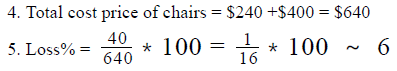Hence, the shopkeeper made a loss of around 6% on the total transaction

QUESTION: 2

### A craftsman sold his identical handcrafted items at a profit of 20 percent. Had he charged 20 percent more per item, his profit per item would have been \$9.6 more. What price did he charge, in dollars, for each item?

Solution:

Given:

• Let the Cost Price of each item be C dollars and the selling price be S dollars
• If Selling Price is 20% more, profit per item is \$9.6 more

To Find: S = ?

Approach:

1. To find the value of S, we need to draft an equation in terms of S. We’re given the relation between Old Profit (when the selling price is S) and the New Profit (when the selling price is 20% greater than S). We’ll use this relation to draft an equation in terms of S.

Working out:

• New Selling Price = S + 20% of S = S + 0.2S = 1.2S
• New Profit = (Old Profit) + \$9.6
• New profit = New SP – CP = 1.2S – C
• Old profit – Old SP – CP = S - C
• So, (1.2S – C) = (S – C) + 9.6
• 0.2S = 9.6
•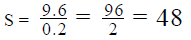Looking at the answer choices, we see that the correct answer is Option D

QUESTION: 3

### The dealer of an electronic store discounted the price of a television and a microwave oven by the same percentage. On which of the two articles did the shopkeeper make a greater profit? (1) The articles were marked up by the same percentage (2) The amount of discount was same for both the articles

Solution:

Step 1 & 2: Understand Question and Draw Inference

• Let’s assume the cost price, selling price and marked price of the television be Ct, St and Mt respectively.
• Let’s assume the cost price, selling price and marked price of the microwave be Cm, Sm and Mm respectively
• Let the discount given be d%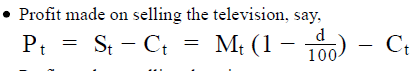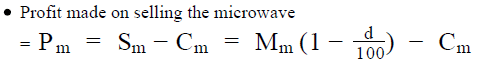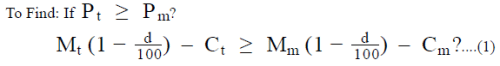Step 3 : Analyze Statement 1 independent

(1) The articles were marked up by the same percentage

• Let the markup% be m%.
• So, writing the markup on television and microwave oven in terms of m%, we get: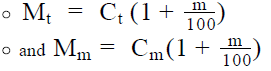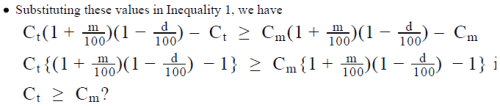We do not know for sure if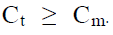Insufficient to answer.

Step 4 : Analyze Statement 2 independent

(2) The amount of discount was same for both the articles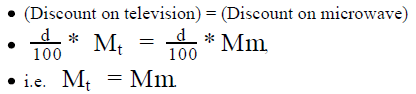• Substituting in inequality (1), we have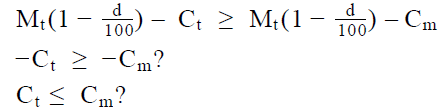We do not know for sure if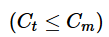Insufficient to answer

Step 5: Analyze Both Statements Together (if needed)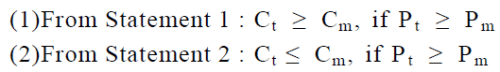Combining both the statements, we have C = C . Hence, the only possible case is Pt = pm . Hence the shopkeeper made equal profit on both the items.

QUESTION: 4

A shoe shop owner lists the price of a pair of shoes as the price at which he purchased the pair from the manufacturer plus a markup
which is equal to 40 percent of the selling price. After some time, he offers a discount on the shoes which is equal to 20 percent of
the selling price. Which of the following best represents the profit, as a percentage of the purchase price, which he earns on selling
the pair of shoes?

Solution:

Given:

• Let the cost price of a pair of shoes be C, the selling price be S and the marked price be M
• Discount offered = 20% of S = 0.2S
• Mark-up = 40% of S = 0.4S
• And, M = C + 40% of S
• M = C + 0.4S

To Find: Profit earned as a % of C

Approach:

1. Required profit %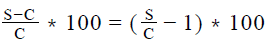• So, to answer the question, we need to find the ratio S/C
2. Now, we are given one equation that links M, C and S. In this equation, if we can substitute M in terms of C or S, we’ll get a linear equation that relates C and S, and so, from that equation, we’ll be able to find the required ratio.
3. So, let’s now look at how we can express M in terms of S or C. We’re told the value of Discount in terms of S.

Using the fact that Discount = M – S, we will be able to express M in terms of S

Working out:

• Expressing M in terms of S
• Given: Discount = 0.2S
• So, M – S = 0.2S
• Therefore, M = 1.2S
• Finding the ratio S:C
• Given: M = C + 0.4S
•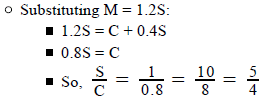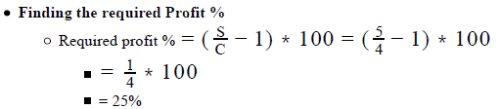Looking at the answer choices, we see that the correct answer is Option E

QUESTION: 5

A shopkeeper offers two successive discounts of 20% each on a sweater and still makes a profit of 60%. By what percentage did
the shopkeeper mark-up the price of the sweater?

Solution:

Given:

• Successive discounts (d and d ) offered by the shopkeeper on a sweater
• d = 20%
• d = 20%
• Profit % of the shopkeeper = 60%

To Find: Mark-up% on the sweater?

Approach:

1.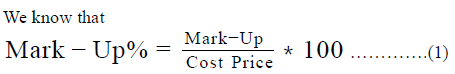• So, in order to know the MarkUp %, we need to know either the ratio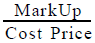or the values of Markup (the amount of markup) and the Cost Price. Since we are not given the dollar values of anything (like cost price, selling price, marked price, discount or profit), we will not be able to find the dollar values of MarkUp and Cost Price. So, we should try to find the ratio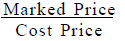2.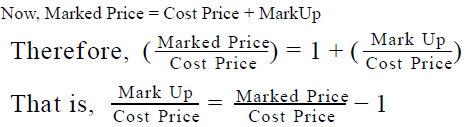• So, in order to find the ratio we need to find the ratio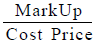we need to fid the ratio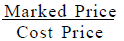3. We are given the discounts availed on the sweater. Using these discounts, we will be able to find the ratio of Marked Price and Selling Price of the sweater.
• Let’s assume the Marked Price to be x.
• So, Marked Price after applying discount d1 = x – d1 % of x
• Now, Marked Price after applying discount d2 = (x – d1% of x) – d2 % of (x – d1 % of x)
So, the selling price of the sweater = (x – d1 % of x) – d2 % of (x  – d1 % of x)
• So,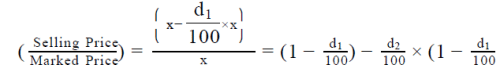We are also given the profit % on the sweater. Using this, we will be able to find the ratio of Selling Price and Cost Price of the
sweater

Selling Price = Cost Price + Profit
Also, we know that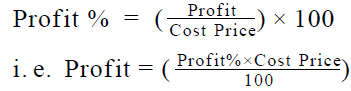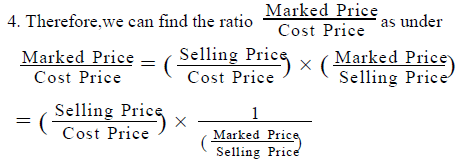Working out: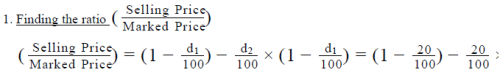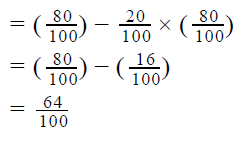2. Calculating the Cost Price
a. Selling Price = 0.64x
b. Profit% = 60%
c. So, we can write 0.64x = CP + 60% of CP, which gives us CP = 0.4x

3. Calculating Mark-Up%
a. Hence, the price of sweater was marked up by = x – 0.4x = 0.6x. So, using (1), the mark-up5 can be given by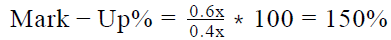QUESTION: 6

A furniture retailer bought 100 identical chairs at a cost of \$20 each. He sold some of the chairs within the first week for \$35 each. In the second week, he sold all the remaining chairs for \$30 each. If his total profit on the sale of the chairs was greater in the second week than in the first week, which of the following can be the number of chairs sold by the retailer in the second week?

I. 30
II. 60
III. 80

Solution:

Given:

• Number of chairs bought by retailer = 100
• Cost price of 1 chair = \$20
• First Week
• Let the number of chairs sold in the first week be z.
• Selling price per chair = \$35
• So, profit per chair = \$35 - \$20 = \$15
• So, total profit on selling z chairs = \$15z
• Second Week
• The number of chairs sold =100 – z
• Selling price per chair = \$30
• So, profit per chair = \$30 - \$20 = \$10
• So, total profit on selling 100 - z chairs = \$10(100 – z) =\$(1000-10z)
• Profit in 2nd week > Profit in 1st week

To Find: Which of the 3 values is/are possible for 100 –z?

Approach:

1. To answer the question, we need to find the possible values of z
2. We’ve already inferred above the profit of 1 and 2 weeks in terms of z.

We’re also given a relation between the profit of 1 and 2 weeks. We’ll use this relation to find the possible values of z.

Working out: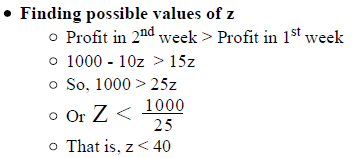• Finding possible values of 100 –z
• Since z < 40, -z > -40
• So, 100-z > 100 – 40
• That is, number of chairs sold in the 2 week > 60
• Evaluating the 3 given values
• Of the 3 given values, the only value that is greater than 60 is the 3 value (80)

Looking at the answer choices, we see that the correct answer is Option C

QUESTION: 7

A shopkeeper purchased 20 identical units of an item at a cost of \$20 per unit. He sold some of the units at a price of \$x per unit and the remaining units at \$y per unit. What was his gross profit made by selling the 20 units of the item?
(1) The number of units sold at \$x per unit was 12
(2) The total revenue generated by selling the 20 units was \$880

Solution:

Step 1 & 2: Understand Question and Draw Inference

Given:

• Total number of units = 20
• Cost price per unit = \$20
• Let the number of items sold at \$x per unit be a
• So, the number of items sold at \$y per unit = 20 – a

To find: Gross Profit

• Gross Profit = (Total Revenue) – (Total Cost)
• = {ax + (20-a)*y } – 20*20
• So, in order to answer the question, we need to find the Total Revenue, and to find the Total Revenue, we need to know a, x and y

Step 3 : Analyze Statement 1 independent

Statement 1 says that ‘The number of units sold at \$x per unit was 12’

• So, a = 12
• However, we do not yet know the value of x and y
• So, Statement 1 is not sufficient to find ‘Total Revenue’ and hence, to get to the answer

Step 4 : Analyze Statement 2 independent

Statement 2 says that ‘The total revenue generated by selling the 20 units was \$880’

• Since we now know the value of ‘Total Revenue’, we can find Gross Profit
• So, Statement 2 is sufficient to answer the question

Step 5: Analyze Both Statements Together (if needed)

Since we’ve already arrived at a unique answer in Step 4, this step is not required

QUESTION: 8

For a manufacturer, the cost of producing x units is given the expression y +kx, where y and k are constants. If the manufacturer sells 100 units at \$50 each, his per unit profit for these 100 units is equal to 64 percent of the selling price per unit and if he sells 250 units at \$40 each, his per unit profit for these 250 units is equal to 70 percent of the selling price per unit. How much profit in dollars would the manufacturer make if he sold 50 units at \$70 each?

Solution:

Given:

• Cost of Producing x units = y +kx, where y and k are constants
• If number of units sold = 100
• Selling Price of each unit = \$50
• Profit per unit = 64% of selling price per unit =64% of 50 =\$32
• If number of units sold = 250
• Selling Price per unit = \$40
• Profit per unit = 70% of the selling price per unit = 70% of 40 = \$28

To Find: Profit made on selling 50 units at \$70 each

Approach:

1. Profit made on selling 50 units at \$70 each = Total Selling price of 50 units – Total cost price of 50 units
• We are given the selling price per unit = \$70. So, we can calculate the total selling price of 70 units
• Cost of producing 50 units = y +kx. We know x = 50. So, we need to find the value of y and k
• As we need to find the value of 2 variables, we would need 2 equations.
2. Finding values of y and k
• We are given the selling price of each of the 100 units and the profit per unit made on selling these 100 units. So, using the formula
• Selling Price = Cost Price + Profit, we can find the cost price of 100 units.
• Also, we know that cost price of producing 100 units = y +100k…….(1)
• Similarly, we are given the selling price of each of the 250 units and the profit per unit made on selling these 250 units. So, using the formula
• Selling Price = Cost Price + Profit, we can find the cost price of 250 units.
• Also, we know that cost price of producing 250 units = y +250k…….(2)
• Thus, we have 2 equations through which we can find the values of y and k.
3. Once, we know the values of k, we can find the cost price of producing 50 units, which can then be used to find the profit made on selling 50 units at \$70 each.

Working out:

1. Selling price of 100 units at \$50 each = 100*50 = \$5000
• Profit per unit = \$32 (analysed in the Given section)
• Profit on 100 units = 100*32 = \$320
• So, Cost price of 100 units = \$5000 - \$3200 = \$1800
• As per the given formula for Cost Price, Cost of producing 100 units = y +100k
• Therefore, y+100k = \$1800……..(1.1)
2. Selling price of 250 units at \$40 each = 250*40 = \$1000
• Profit per unit = \$28 (analysed in the Given section)
• i. Profit on 250 units = 250*28 = \$7000
• So, Cost price of 250 units = \$10000 - \$7000 = \$300
• As per the given formula of cos tprice, Cost of producing 250 units = y +250k
• Therefore, y + 250 - k = \$3000……..(2.2)
3. Solving equations (1.1) and (2.2), we have y = 1000 and k = 8
4. So, cost of producing 50 units = y +50k = 1000 +50*8 = \$1400
• Selling price of 50 units = 50*70 = \$3500
• Hence, profit on selling 50 units at \$70 each = \$3500 - \$1400 = \$2100

Thus, the manufacturer made a profit of \$2100 on selling 50 units at \$70 each.

QUESTION: 9

If the maximum discount that a merchant who doesn’t want to sell an item at a loss can offer on that item is 20%, what will be the
profit percentage that the merchant makes if he sells the item at its marked price?

Solution:

Given:

• Let Cost Price of the item be C, Marked Price be M and Selling Price be S
• Maximum discount for no loss = 20%
• This means, At 20% discount, the item is sold at Zero Profit, Zero Loss
• That is, At 20% discount, the discounted price of the item is equal to the Cost Price

To Find: % Profit if the item is sold at its Marked Price

Approach:

1.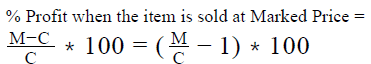• So, to answer this question, we need to find the ratio M/C
2. We are given one relation between M and C : At 20% discount on M, the discounted price is equal to the cost price. Using this relation, we’ll find the required ratio.

Working out:

• (Value of 20% Discount) = 20% of M = 0.2M
• This is the maximum discount that the merchant can offer without going into loss
• This means, at this point, his profit is zero (if the selling price was any lesser, he would make a loss)
• So, at this discount, his selling price is equal to his cost price
• Now, (Selling price at 20% discount on M) = M – (Value of 20% Discount) = M – 0.2M = 0.8M
• So, 0.8M = C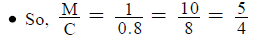• So, Required % Profit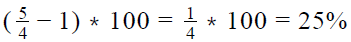Looking at the answer choices, we see that the correct answer is Option C

QUESTION: 10

A retailer purchases an item at \$700 per piece and sells it at a profit of 30 percent. To encourage the sale of the item in the holiday season, the retailer plans to offer at least two discount coupons to his loyal customers. At the same time, he wants to make sure that his profit margin does not fall below 10 percent. If the customers makes use of the discount coupons in an order which maximizes their discount, which of the following coupons should the retailer offer?

I. \$50 off
II. 8% off
III. 10% off

Solution:

Given:

• Cost Price of the item, CP = \$700
• Profit % before offering discounts = 30% (of CP)
• So, Profit = 30% of 700 = 210
• Therefore,
• Selling Price before offering discounts, SP = CP + Profit = = 700 + 210 = \$910
• Minimum required Profit = 10%
• = 10% of 700 = \$70
• 2 or more discount coupons are offered
• Discount coupons used in the order that maximizes the discount

To Find: Which of the given 3 discount coupons should the retailer offer?

Approach:

1. To determine which discount coupons can be offered, we need to analyze the constraint on discount.
• The maximum discount allowed is one that leads to the minimum required profit (10%)
2. So, we’ll calculate the value of the maximum discount allowed.
• Minimum Profit = (Minimum Required Profit %)*(CP)
• Profit = SP – CP
• So, Profit is minimum when SP is minimum
• So, Minimum SP = CP + (Minimum Profit)
• Now, SP = Marked Price – Discount
• So, SP is minimum when discount is maximum
• Therefore, (Maximum Discount) = (Marked Price) – (Minimum SP)
3. Next, we’ll evaluate all possible pairs that can be made from the given 3 discount coupons to determine the pairs in which the discount availed by using the discount coupons is greater than the maximum discount allowed. These are the pairs of discount coupons that cannot be offered by the retailer.

4. Finally, by looking at the pairs of discount coupons that cannot be offered by the retailer, we’ll determine the discount coupons that cannot be offered by the retailer.

• For example, if pairs A-B and B-C cannot be offered but pair A-C can be offered, this means it is discount coupon B that should not be offered by the retailer.

Working out:

• Finding the maximum discount allowed
• As we have seen above, minimum profit = \$70
• This is the case of maximum discount
• In the case of maximum discount, Selling Price = 700 + 70 = \$770
• Whereas, the Selling Price beforre offering the disocunts = \$910
• So, the maximum discount allowed = \$910 - \$770 = \$140
• So, we need to ensure that any combination of 2 coupons from among the 3 given doesn’t lead to a discount greater than \$140

• Evaluating the possible combinations of given discount coupons
• 3 pairs are possible out of the 3 coupons- let’s discuss the discount in each:
• Case  1 : \$ 50 off, 10% off
• Here, to maximize the discount, a person will avail the % discount first and then the cash discount.
• So the maximum discount that can be availed using these 2 coupons is: 10% of 910 + 50 = 91 + 50 = 141 dollars, which is greater than \$140
• So, the retailer should not offer \$50 off and 10% off coupons together because then customers will avail a discount greater than \$140
• Case  2 : \$ 50 off, 8% off
• Here again, to maximize the discount, a person will avail the % discount first and then the cash discount
• So, the Maximum discount that can be availed using these 2 coupons = 8% of 910 + 50 = 72.8 + 50 = \$122.8, which is less than \$140
• So, these 2 coupons together do not hurt the retailer’s minimum profit margin
• Case  3 : 10% off, 8% off
• In this case, the final Selling Price = 910\$*(1 – 0.1)(1-0.08) = 910*0.9*0.92 = \$753
• So, Discount availed = \$910 – \$753 = \$157
• This is again greater than \$140. So, this combination again cannot be offered.
• Thus, we see that the following 2 combinations cannot be offered:
• \$50 off, 10% off
• 10% off, 8% off
• But the following combination can be offered:
• \$50 off, 8% off
• This means, the retailer should not offer the 10% off discount coupon. He can offer \$50 off and 8% off discount coupons.

Looking at the answer choices, we see that the correct answer is Option A

QUESTION: 11

If a man sells x oranges for a dollar, he loses 45 percent. By how much percentage should the man reduce his cost to make a profit
of 10 percent on selling 2x oranges in a dollar?

Solution:

Given:

x oranges are sold for \$1 at a loss of 45%
To Find: The percentage by which the cost should be reduced so that 2x oranges are sold for \$1 at a profit of 10%

1. ApproLet’s assume the cost price of x oranges when the man sells the x oranges for \$1 and loses 45% be CP1 and the cost price of x oranges when he sells 2x oranges for \$1 be CP2ach:
• So, the percentage by which the person should reduce his cost be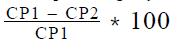2. Calculating CP1
a. Selling price of x oranges = \$1
b. Loss% = 45%
c. Now, we know that Selling Price = Cost Price - Loss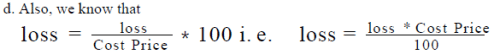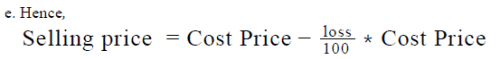f. As we know the selling price and the loss %, we can find the Cost Price of x oranges, i.e. CP1

2. Calculating CP2
a. Selling price of 2x oranges = \$1
b. Profit% = 10%
c. Now, we know that Selling Price = Cost Price + Profit…..(3)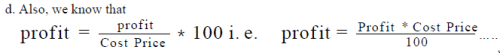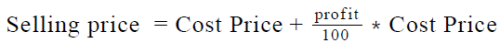So, we can find the Cost Price of 2x oranges.
g. Hence, we can find the cost price of x oranges i.e. CP2

Working out: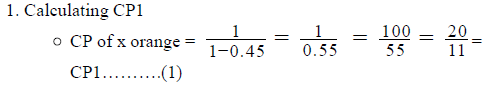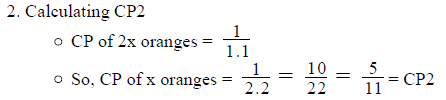3. The percentage by which the man should decrease his price =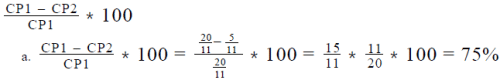Thus the man should reduce his price by 75% to make a profit of 10% on  selling 2x oranges for a dollar.

QUESTION: 12

Jane wants to purchase a history book and a literature book that are sold in regular book shops at \$20 and \$30 respectively. The same books are also available in an online used-book store at discounts of 20% and 30% respectively on their respective regular book shop prices. At the time of billing, if the number of books purchased is 2 or more, the online used-book store provides an additional discount of 10% on the sum of discounted prices of purchased books, and finally, adds \$2.7 to the bill as shipping charges. The amount saved by Jane by purchasing the books from the used-book store will be what percentage of the amount she would have paid for the two books at a regular book shop?

Solution:

Given:

• Regular Book Shop
• Price of History book = \$20
• Price of Literature book = \$30
• Online used-book store
• Discount on History book = 20%
• Discount on Literature book = 30%
• Additional discount = 10% of (Discounted Price of History Book + Discounted Price of Literature Book)
• Note that this additional discount applies since Jane bought 2 books
• Shipping charges = \$2.7

To Find: % Savings of Jane by buying the 2 books from online used-book store.

Approach:

1.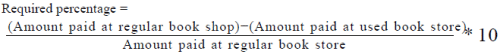• So, to answer the question, we need to know ‘amount paid at used
book store’ and ‘amount paid at regular book shop’
2. (Amount paid at used-book store) = (Discounted Price of History Book + Discounted Price of Literature Book) – (Additional Discount) + (Shipping Charges)
3. (Amount paid at regular book shop) = (Price of History Book) + (Price of Literature Book)

Working out:

• Finding Amount paid at used-book store
• Discount on History Book = 20% of 20 = 4
• So, Discounted price of History book = 20 - 4 = \$16
• Discount on Literature book = 30% of 30 = 9
• So, Discounted price of Literature book = 70% of 30 = \$21
• Discounted Price of History Book + Discounted Price of Literature Book = \$16 + \$21= \$37
• So, additional discount = 10% of \$37 = \$3.7
• So, (Amount paid at used-book store) = \$37 - \$3.7 + \$2.7 = \$36
• Finding Amount paid at regular book shop
• (Amount paid at regular book shop) = \$20+ \$30 = \$50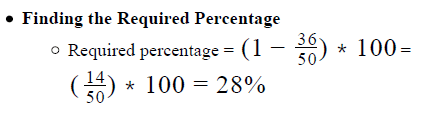Looking at the answer choices, we see that the correct answer is Option A

QUESTION: 13

An entrepreneur who is planning to set up a restaurant estimates that the monthly costs of running the restaurant will be given by the formula C = 15000 + 2000n + 5m, where C is in dollars, n is the number of employees at the restaurant and m is the number of orders received in the month. He plans to start the restaurant with only 2 employees and to hire 1 new employee each month. He estimates that the average order value at his restaurant will be \$50 and that the number of orders per month will increase by 10% month on month. If the estimated number of orders the restaurant receives in the first month of its existence is 1000, then as per this model, after how many months of operation will the restaurant first achieve a profit margin of 20% or more?

Solution:

Given:

• Monthly costs C = 15000 + 2000n + 5m, where
• n = number of employees
• m = number of orders
• Average order value = \$50
• Month 1:
• n = 2
• m = 1000 (estimated)
• Month 2:
• n = 2 + 1 = 3
• m = 1.1*1000 (estimated)
• In general, for kth Month:
• n = 2 + (k – 1)
• m = (1.1) *1000

To Find: After how many months of operation is Profit ≥ 20%

Approach:

1.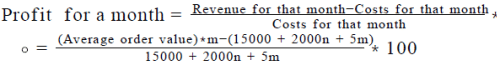2. We’re given the month-on-month growth trend for both n and m. So, we can find the value of n and m for all months of operation. We also know the Average order value and it is constant for all months of operation. So, starting from the 1 month, we’ll find the value of Monthly Profit % till we get to the month for which the Profit % is greater than or equal to 20%

Working out: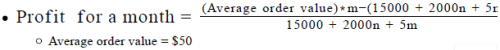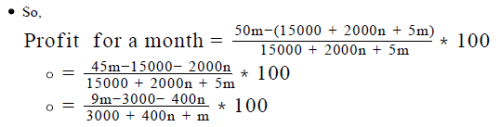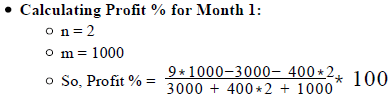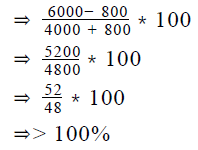• Thus, we see that the profit for the 1 month itself is estimated, as per the given model, to be greater than 100%
• Therefore, we do not need to do the evaluations for any more months. The profit margin is (much) greater than 20% in Month 1 itself.

Looking at the answer choices, we see that the correct answer is Option A

QUESTION: 14

Sandy bought a share for \$100. At what price should he sell the share to make a profit that is equal to 50 percent of the selling
price?

Solution:

Given:

• Cost Price of the share = \$100
• Profit to be made = 50% of the selling price
• Let the selling price be S

To Find: Value of S?

Approach:

• We know that Selling Price = Cost Price + Profit
• As we know that Profit = 50% * Selling Price and we know the value of cost price we can calculate the value of the selling price using this equation.

Working out:

1. S = Cost Price + 50% * S
• S = \$100 + 0.5S
• 0.5S = \$100
• S = \$200

So, Sandy should sell the share at \$200

Correct Option:D

QUESTION: 15

A manufacturer produces items at a fixed monthly cost of \$2000 plus a variable cost of \$100 per item. If he needs to sell N items to make a monthly profit of \$50 per item, what is the value of N?
(1) Each item produced is sold for \$200.
(2) If the fixed cost is not included in the cost and the manufacturer still sells N items at the same selling price, he will make a monthly profit of \$100 per item.

Solution:

Step 1 & 2: Understand Question and Draw Inference

Given:

• Fixed Cost = \$2000
• Variable Cost = \$100 per item

To find: N ( = Number of items to be sold to make a profit of \$50 per item)

• Let the selling price each item be S dollar
• So, revenue generated by selling N items = N * S
• Cost of producing N items = N * 100 +\$2000
• Profit on selling N items = N * S – (N * 100 +\$2000)
• 50* N = N* S – ( N* 100 +\$2000)
• So, we need to find the value of S

Step 3 : Analyze Statement 1 independent

Statement 1 says that ‘Each item produced is sold for \$200

• So, S = \$200
• As we know the value of S we can find the value of N.

Step 4 : Analyze Statement 2 independent

Statement 2 says that ‘If the fixed cost is not included in the cost and the
manufacturer still sells N items at the same selling price, he will make a
monthly profit of \$100 per item’

• S = Variable Cost per item + \$100
• S = \$100 + \$100 = \$200
• As we know the value of S, we can calculate the value of N.

So, Statement 2 is sufficient to answer the question

Step 5: Analyze Both Statements Together (if needed)

Since we’ve already arrived at a unique answer in Steps 3 and 4, this step is not required

QUESTION: 16

A local grocer purchased eggs at a cost of c dollars per dozen each morning and sold all of them by the day end at a cost of s dollars per dozen. He made a profit of 100% on the sale of eggs each day. One morning there was a minor accident at the grocer’s shop in which some of the eggs he had bought that morning broke and therefore, were rendered unsaleable. The grocer sold the remaining eggs at their usual selling price and made a profit of 50% on the sale of eggs that day. What percentage of the eggs bought by the grocer that morning broke in the accident?

Solution:

Given:

• Cost price of eggs = c dollars per dozen
• Selling price of eggs = s dollars per dozen
• % profit = 100%
• This is the profit when all the eggs that were purchased get sold
• On the day of the accident,
• Let eggs bought = x dozen
• Eggs broken = b dozen
• So, eggs sold = x – b dozen
• % profit when b eggs broke, that is when only x – b dozen eggs get sold = 50%

To Find: % of eggs that broke = ?

Approach:

1.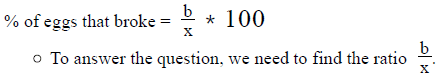2.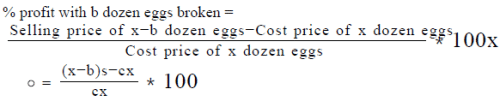•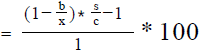• We know the value of % profit with b dozen eggs broken. So, if we know the value of s/c then, by using the above equation, we’ll be able to get the ratio b/x
3.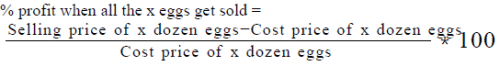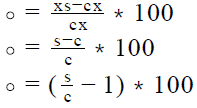• We know the value of % profit when all the x eggs get sold. So, by equating the above expression to this value, we’ll be able to get the ratio s/c

Working out:

• Finding the ratio s/c
• The % profit when all the x eggs get sold is 100%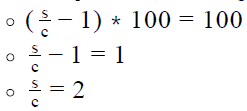• Finding the ratio b/x
• % profit with b dozen eggs broken = 50%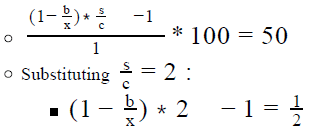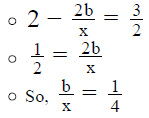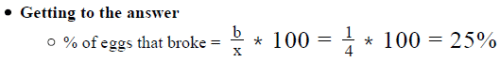Looking at the answer choices, we see that the correct answer is Option A

QUESTION: 17

Rafael spent his pocket money of \$150 to buy three different articles A, B and C from the same shopkeeper. Rafael used 30 percent of his pocket money to buy article A, which was discounted by 10 percent, 30 percent of his pocket money to buy article B, which was discounted by 25 percent and the rest of the money to buy article C, which was discounted by 50 percent. If articles A, B and C were marked up by 25 percent, 100 percent and 140 percent respectively, what was the percentage profit made by the shopkeeper on selling the articles?

Solution:

Given:

• Money available with Rafael = \$150
• Rafael buys 3 items A, B and C, from the same shopkeeper, the details of
• which are as below: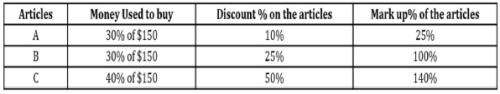To Find: Profit % of the shopkeeper on selling the articles A, B and C.

• Now, we know that Profit% =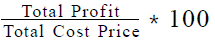• Total Profit = Profit made on Article A + Profit made on article B + Profit made on article C
• Total Cost Price = Cost Price of Article A + Cost Price of article B + Cost Price of article C
• So, we need to find the profit made on each article and the cost price of- each article.

Approach:

1. To Find the profit made on each article, we need to find the following:
• Selling price of the article = Amount spent by Rafael on buying the article
• Cost Price of the article

i) We are given the selling price, the discount% and the mark-up% of the articles. We will use this information to find the cost price of each article.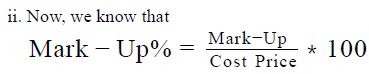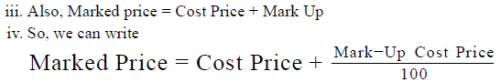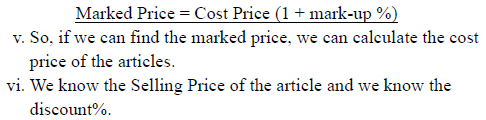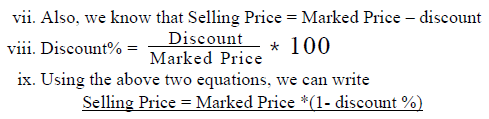x. So, we can find the Marked Price using the above relation

After calculating the selling price and cost price of an article, we can calculate the profit made on the article using the relation Profit = Selling Price – Cost Price

Working out: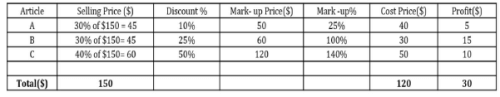Article A :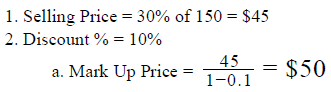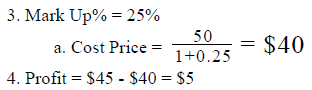Article B :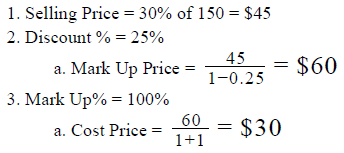4. Profit = \$45 - \$30 = \$15

Article C :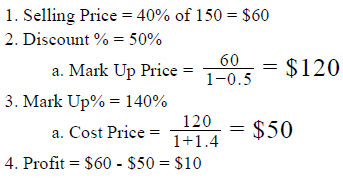Total Profit made by the shopkeeper on the articles = \$5 +\$15 +\$10 = \$30
Total Cost price of the articles = \$40 + \$30 + \$50 = \$120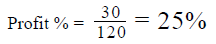QUESTION: 18

A merchant bought an item at a cost of c dollars and marked up its price by 40%. However the item did not sell and the merchant was
forced to discount the price of the item by 20%. What was the percentage profit earned by the merchant by selling the item at the
discounted price?

Solution:

Given:

• Cost Price (dollars) = c
• Markup % = 40% (of c)
• So, (Value of Markup) = 0.4c
• Therefore, Marked Price = Cost Price + Markup = c + 0.4c = 1.4c
• Value of Discount = 20% (of Marked Price)
• So, (Discounted Price) = Marked Price – Value of Discount = Marked Price – 20% of Marked Price = 80% of Marked Price
• (Selling Price) = (Discounted Price) = 80% of Marked Price

To Find: % Profit earned by selling at Discounted Price

Approach: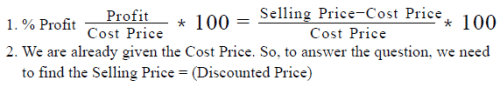Working out: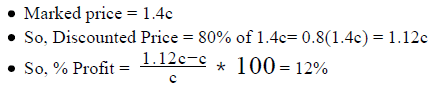Looking at the answer choices, we see that the correct answer is Option B

QUESTION: 19

A shopkeeper buys two printers A and B. Had the shopkeeper paid the full price of both printers, they would have cost the same to him. However, he buys printer A with a down payment of 10 percent of the cost of printer A and printer B with a down payment of 20 percent of the cost of printer B and repays the remaining cost and the individual finance charges of the printers A and B over a period of time. The finance charges for printers A and B are equal to 40 percent of the remainder of the cost of printer A and y percent of the remainder of the cost of Printer B respectively. What should be the value of y so that the cost of buying the two printers is equal for the shopkeeper?

Solution:

Given:

• Marked price = x dollars
• First discount offered = d%
• Additional discount offered = 2d dollars

To Find: Single discount % equivalent to total discount given?

Approach:

1.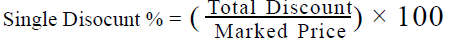• So, in order to find the Single Discount %, we need to know the Total Discount availed by the customer
2. We know that
• (Selling Price) = (Marked Price) – (Total discount)
• So, (Total Discount) = (Marked Price) – (Selling Price)
• Therefore, once we know the Selling Price, we’ll be able to find the Total Discount.
3. Now, we can calculate the selling price of the article by applying the discount% and the dollar discounts.

Working out: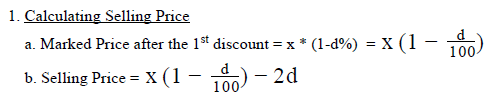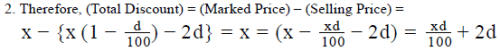3. So, we can write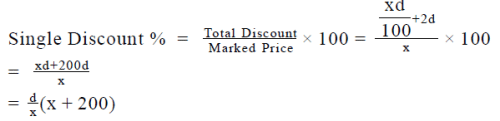QUESTION: 20

A light bulb manufacturer ships 10 boxes consisting of 100 light bulbs each to a retailer. The manufacturer bills the retailer at a rate of \$10 per bulb with a condition that the defective light bulbs may be returned to the manufacturer for full refund. The probability of a light bulb in a box being defective is 20 percent. If the manufacturer makes a profit of 100 percent on each non-defective light bulb and he has to bear a return shipment cost of \$2.5 per defective light bulb, which he treats as lost revenue, what is the profit percentage of the manufacture on the whole transaction?

Solution:

Given:

• Number of light bulbs in a box = 100
• Number of boxes shipped = 10
• So, Total light bulbs shipped = 10*100 = 1000
• Selling price of each non-defective light bulb = \$10
• Profit % on each non-defective light bulb = 100%
• Probability(Defective Light Bulb) = 0.20
• Return shipment cost of each defective light bulb = \$2.50

To Find: Total Profit % of the manufacturer?

Approach:

1.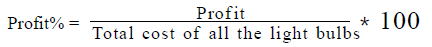• So, to find the profit% of the manufacturer, we need to find the profit made by the manufacturer and the total cost incurred by the manufacturer
• Profit = Total net revenue – Total cost of all the light bulbs
• Thus we need to find the total net revenue and the total cost of all the light bulbs.
• For finding the total net revenue and the total cost of all the light bulbs, we need to find first the number of defective and non-defective light bulbs.
2. Calculating number of Defective and Non-defective light bulbs
• As we know the total number of bulbs shipped and the probability of a bulb being defective, we can find the number of defective bulbs as Total defective light bulbs = Total light bulbs shipped
• Once, we find the number of defective light bulbs, we can find the number of non-defective light bulbs a Total non − defective light bulbs = Total light bulbs
3. ​Total Net Revenue Calculation

a. Hence the total net revenue of the manufacturer can be calculated as

Total Net revenue=(Total revenue from non-defective light bulbs)- (Total lost revenue from defective light bulbs)

b. As we know the selling price of each non-defective light bulb and the number of non-defective light bulbs, we can calculate the total
revenue of the manufacturer from the non-defective light bulbs as

Total Revenue from non − defective light bulbs

c. Also, the manufacturer has to recognise the shipment charges of the defective bulbs as lost revenue, it can be calculated as

4. Total Cost Calculation

• Total cost of all the light bulbs = Total light bulbs * Cost Price of each light bulb
• Please note here that we are calculating the cost price on total light bulbs as the transaction involves producing both the defective and non-defective light bulbs
• As we are given the selling price and profit % on each light bulb, we can calculate the cost price of each light bulb as

Selling Price = Cost Price + Profit ..............(1)

Also, we know that,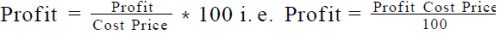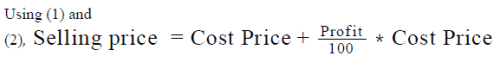Working out:

1. Calculating number of Defective and Non-defective light bulbs

a. Total number of bulbs shipped = 1000
b. Total defective light bulbs = 0.2 * 1000 = 200
c. So, total non-defective light bulb = 1000 – 200 = 800

2. Total Net Revenue Calculation
a. Selling price of each non-defective light bulb = \$10
b. Total revenue on the non-defective light bulbs = 800 * 10 = \$8000
c. Lost revenue for each defective light bulb = \$2.5
d. So, lost revenue for total defective bulbs = 2.5 * 200 = \$500
e. Hence total net revenue = \$(8000 – 500) = \$7500

3. Total Cost Calculation
a. Selling price of each non-defective light bulb = \$10
b. Profit % on each non-defective light bulb = 100%
c. Cost price of each light bulb = \$5
d. Total cost in producing all the light bulbs = 1000* 5 = \$5000

4. Profit made = \$(7500 – 5000) = \$2500

5. Profit % =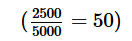%
Hence the manufacturer made a profit of 50% on the whole transaction.Use Code STAYHOME200 and get INR 200 additional OFF Use Coupon Code